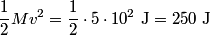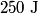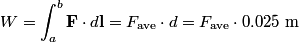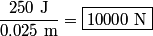## Solution to 1986 Problem 8

 The kinetic energy of the stone just before it hits the nail is\begin{align*}\frac{1}{2} M v^2 = \frac{1}{2} \cdot 5 \cdot 10^2 \mbox{ J} = 250 \mbox{ J}\end{align*}By the time the stone comes to rest, it has lost all of its kinetic energy. This means that the nail has done$250 \mbox{ J}$ of work on the stone.\begin{align*}W = \int_{a}^{b} \mathbf{F} \cdot d \mathbf{l} = F_{\text{ave}} \cdot d = F_{\text{ave}} \cdot 0.025 \mbox{ m}\...So, the answer is\begin{align*}\frac{250 \mbox{ J}}{0.025 \mbox{ m}} = \boxed{10000 \mbox{ N}}\end{align*}So, answer (D) is correct.